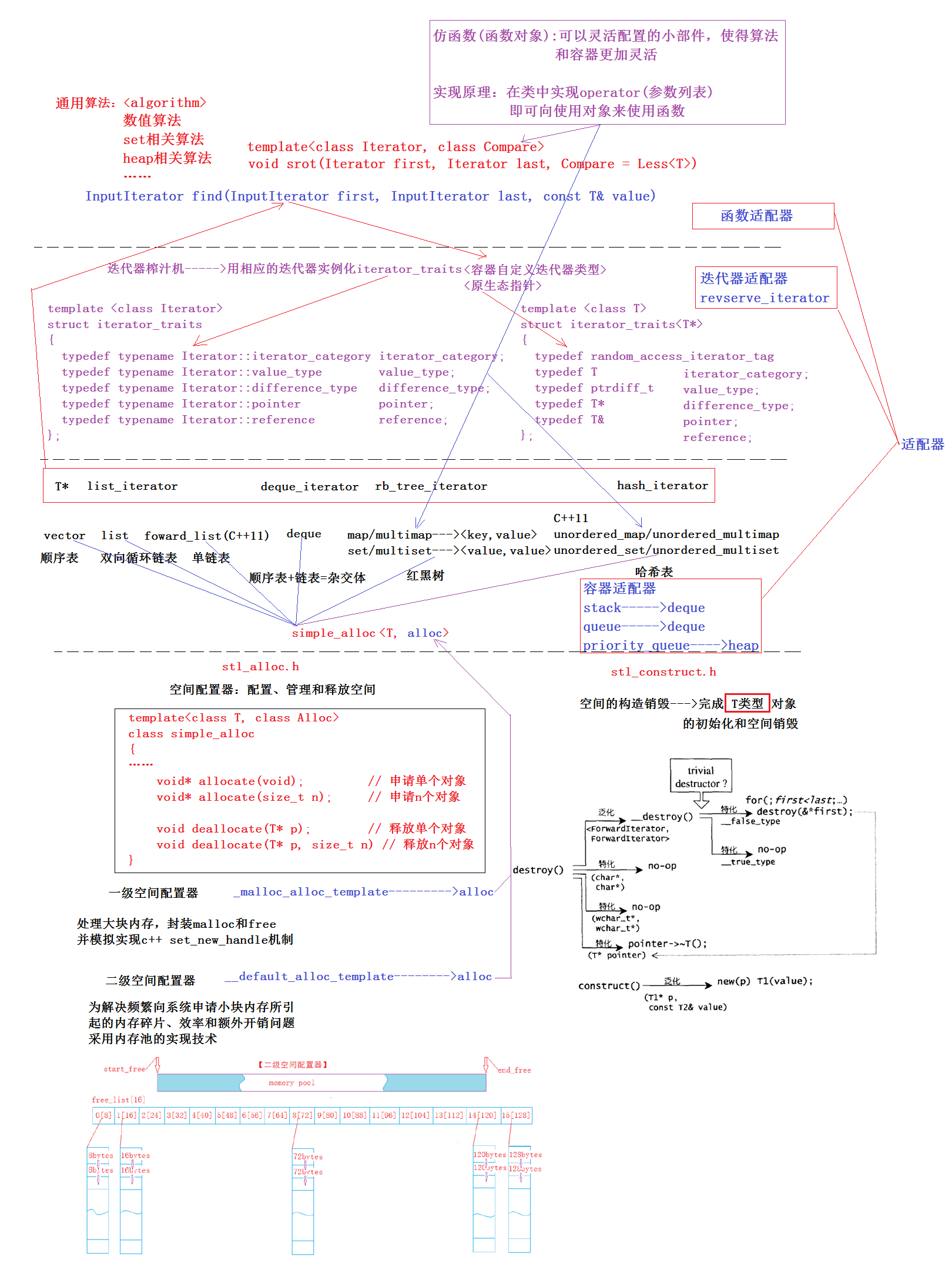## [C++ 系列] 88. STL进阶及简单总结

### 1. STL的本质

`STL` 设计理念：追求代码高复用性以及运行速度的高效率，在实现时使用了许多技术，因此熟悉 `STL` 不仅对我们正常使用有很大帮助，而且对自己的知识也有一定的提高。

### 2. STL的六大组件

#### 2.1 容器

`STL` 中的容器，可以划分为两大类：序列式容器和关联式容器。1. 熟悉每个容器的常用接口以及帮助文档查阅，并能熟练使用，建议再刷题以及写项目时多多应用，熟能生巧
2. 熟悉每个容易的底层结构、实现原理以及应用场景，比如：红黑树、哈希桶
3. 熟悉容器之间的区别：比如vector和list区别？map和set区别？map和unordered_map的区别？

#### 2.2 算法

`STL` 中通用算法总共有70多个，为我们提供了一个 `algorithm` 库，主要包含：排序，查找，排列组合，数据移动，拷贝，删除，比较组合，运算等。 以下只列出了部分常用的算法：

accumulate 元素统计
binary_search 二分查找
copy 拷贝
copy_backward 逆向拷贝
copy_n 拷贝n个元素
count 计数
count_if 在特定条件下计数
equal 判断两个区间相等与否
ﬁll 填充元素
ﬁll_n 填充元素n次
ﬁnd 循环查找
ﬁnd_if 循环查找符合特定条件元素
ﬁnd_end 查找某个子序列的最后一次出现点
ﬁnd_ﬁrst_of 查找某个元素首次出现点
for_each 对区间内的每隔一元素实行某种操作
is_heap 判断某区间是否为一个heap
is_sorted 判断某区间是否已排序
lexicographical_compare 以字典顺序进行比较
max 获取最大值
max_element 最大值所在位置
merge 合并两个序列
min 获取最小值
min_element 最小值所在位置
next_permutation 获取下一个排列组合
pre_permutation 获取前一个排列组合
partial_sort 局部排序
partial_sum 局部求和
partition 分割
remove 删除某类元素
remove_copy 删除某类元素并将结果拷贝到另一个容器中
remove_if 有条件的删除某类元素
replace 替换某类元素
replace_if 有条件的替换
reverse 反转序列
sort 排序(不稳定)
stable_partition 分割并保持元素的相对次序
stable_sort 分割并保持相等元素的相对位置(稳定排序算法)
unique 取出重复性元素
make_heap 创建堆
push_heap 堆插入
pop_heap 堆删除
sort_heap 堆排序

1. 熟悉常用算法的作用，并熟练使用
2. 熟悉房间算法时间复杂&空间复杂度求解方式

#### 2.3 迭代器

##### 2.3.1 什么是迭代器

`C++` 中迭代器本质：是一个指针，让该指针按照具体的结构去操作容器中的数据。

##### 2.3.2 为什么需要迭代器

``````template<class InputIterator, class T>
InputIterator find ( InputIterator first, InputIterator last, const T& value )
{
for ( ;first!=last; first++)
if ( *first==value )
break;

return first;
}
``````

`ﬁnd` 算法在查找时，与具体的数据结构无关，只要给出待查找数据集合的范围，`ﬁnd` 就可在该范围中查找，找到返回该元素在区间中的位置，否则返回 `end`• 只要是容器的迭代器，就一定有 `++` 算法，`*` 解引用算法，这是由它底层迭代器封装方式决定的。
##### 2.3.3 迭代器应该由谁负责提供

``````// vector中：
typedef T* iterator;
iterator begin();
iterator end();
find(v.beign(), v.end(), 5);

// list中
typedef list_iterator<T, T&, T*> iterator;
iterator begin();
iterator end();
find(l.begin(), l.end(), 5);
``````
##### 2.3.4 迭代器实现原理

1. `vector`
• 因为 `vector` 底层结构为一段连续空间，迭代器前后移动时比较容易实现，因此 `vector` 的迭代器实际是对原生态指针的封装，即：`typedef T* iterator`
1. `list`
• `list` 底层结构为带头结点的双向循环链表，迭代器在移动时，只能按照链表的结构前后依次移动，因此链表的迭代器需要对原生态的指针进行封装，因为当对迭代器 `++` 时，应该通过节点中的 `next` 指针域找到下一个节点。可参考：[C++系列] 56. list深度剖析及模拟实现

1. 迭代器能够像指针一样方式进行使用：重载 `reference operator*()` / `pointer operator->()`
2. 能够让迭代器移动
• 向后移动：`self& operator++() / self operator++(int)`
• 向前移动：`self& operator--() / self operator--(int)` (注意：有些容器不能向前移动，比如 `forward_list`)
1. 支持比较-因为在遍历时需要知道是否移动到区间的末尾 `bool operator!=(const self& it)const / bool operator==(const self& it)const`
##### 2.3.5 迭代器与类的融合
1. 定义迭代器类
2. 在容器类中统一迭代器名字

``````// 比如list：
template <class T, class Alloc = alloc>
class list
{
// ...
typedef __list_iterator<T, T&, T*>             iterator;
// ...
};
``````
1. 在容器类中添加获取迭代器范围的接口：

``````template <class T, class Alloc = alloc>
class list
{
// ...
iterator end(){ return node;}
// ...
};
``````

##### 2.3.7 迭代器萃取(了解)

``````#include <iostream>
#include <vector>
#include <typeinfo>
using namespace std;

class A{};

// 希望
template <class T>
struct unknown_class
{
// 待萃取类型
typedef T return_type;
};

/****************************************/

// 容器萃取机
template <class unknown_class>
struct unknown_class_traits
{
// 在此必须加上typename
// 因为unknown_class不知道其为类型、函数、变量
// 更不知道return_value为类型、函数、变量
// 所以显示加上typename否则编译器不知道后面为类型
typedef typename unknown_class::return_type return_type;

// 萃取vector传入value_type即可
// typedef typename unknown_class::value_type return_type;
};

// 模板与模板函数
// 加入typename显示声明其为类型
template <class unknown_class>
typename unknown_class_traits<unknown_class>::return_type
func(unknown_class u)
{
typedef typename unknown_class_traits<unknown_class>::return_type return_type;
return return_type();
}

int main()
{
// 需要函数将unknown_class中的类型萃取出来定义变量
unknown_class<A> a;
// unknown_class<int> a;
// vector<int *> a;

// 若b的类型为能与a相同那么类型萃取成功
auto b = func(a);

cout << typeid(b).name() << endl;
return 0;
}
``````

#### 2.4 适配器

`STL` 中适配器总共有三种类型：

• 容器适配器 `stack``queue`
`stack` 的特性是后进先出，`queue` 的特性为先进先出，该种特性 `deque` 的接口完全满足，因此 `stack``queue` 在底层将 `deque` 容器中的接口进行了封装。

``````template < class T, class Container = deque<T> >
class stack { ... };

template < class T, class Container = deque<T> >
class queue { ... };
``````

#### 2.5 仿函数

``````#include <vector>
#include <algorithm>
class Mul2
{
public:
void operator()(int& data)
{ data <<= 1;}
};

void mul2(int & val)
{
val *= 2;
}

class Mod3
{
public:
bool operator()(int data)
{ return 0 == data % 3;}
};

int main()
{
// 给容器中每个元素乘2
vector<int> v{1,2,3,4,5,6,7,8,9,0};
// 临时对象
for_each(v.begin(), v.end(), Mul2());

// 也可显示定义对象
Mul2 m2;
for_each(v.begin(), v.end(), m2);

// 也可直接传函数
for_each(v.begin(), v.end(), mul2);

// 上三者等价，将扩大2*2*2=8倍
for (auto e : v)
cout << e << " ";
cout << endl;

// 删除容器中3的倍数
auto pos = remove_if(v.begin(), v.end(), Mod3());
v.erase(pos, v.end());

// 将容器中的元素打印出来
// 注意：对于功能简单的操作，可以使用C++11提供的lambda表达式来代替
// lambda表达式实现简单，其在底层与仿函数的原理相同，编译器会将lambda表达式转换为仿函数
for_each(v.begin(), v.end(), [](int data){cout << data << " "; });
cout << endl;
return 0;
}
``````

### 3. STL框架